7.
(expires 2/18)     Fit the points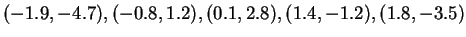by means of a quadratic function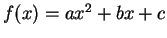, using the least square method. First, do this step by step, as we did in class; then, use the built-in Maple command, described in the notes. Check that the two solutions agree.

8.
(expires 2/18)     Fit the set of points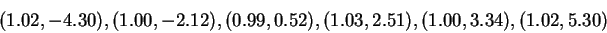with a line, using the least square method we used in class. You will see that this is not a good fit. Think of a better way to do the fit and use Maple to do it. Explain in your solution why you think your better way is better.

9.
(expires 2/18)     In this problem we will estimate the charge of the electron: If an electron of energy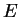is thrown into a magnetic field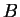, perpendicular to its velocity, its trajectory will be deflected into a circular trajectory of radius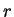. The relation between these three quantities is: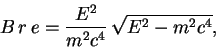(1)

where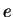and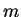are, respectively, the charge and the mass of the electron, and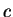is the speed of light. The rest mass of the electron is defined as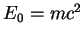, and is about equal to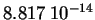Joules. In our experimental set-up the energy of the emitted electrons is set to be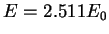.
Use read to make Maple load and execute the commands in the file electron_data.txt, which is located in the Worksheets directory of the mat331 account. This defines a list called electron. Each element of the list is a pair of the form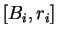, and these quantities are expressed in Teslas and meters. Use least square fitting to determine the best value for. [Hint: Notice that the right hand side of (1) is just a constant--calculate it once and for all and give it a name. Then (1) is a very easy equation, which is linear in the unknown parameter. To verify your solution:Coulomb].
Physical constants courtesy of N.I.S.T.

10.
(expires 2/18)     Prove relation (1), knowing the following physical facts: In relativistic dynamics Newton's law is replaced by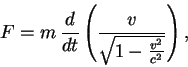(2)

where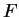is the force acting on a particle,its mass and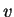, a function of time, its velocity. In the case at hand, the force exerted by a magnetic fieldon an electron is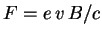. Recall that in a circular motion the acceleration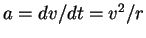,being the radius of the circle. Since (1) is expressed in terms of the energy, rather than the velocity, you also need Einstein's formula,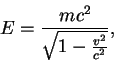(3)

which can be solved in terms of.

MAT 331
2002-02-10